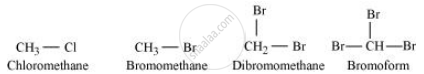Share

# Arrange each set of compounds in order of increasing boiling points Bromomethane, Bromoform, Chloromethane, Dibromomethane. - Chemistry

#### Question

Arrange each set of compounds in order of increasing boiling points

Bromomethane, Bromoform, Chloromethane, Dibromomethane.

#### Solution 1For alkyl halides containing the same alkyl group, the boiling point increases with an increase in the atomic mass of the halogen atom.

Since the atomic mass of Br is greater than that of Cl, the boiling point of bromomethane is higher than that of chloromethane.

Further, for alkyl halides containing the same alkyl group, the boiling point increases with an increase in the number of halides. Therefore, the boiling point of Dibromomethane is higher than that of chloromethane and bromomethane, but lower than that of bromoform.

Hence, the given set of compounds can be arranged in the order of their increasing boiling points as:

Chloromethane < Bromomethane < Dibromomethane < Bromoform.

#### Solution 2

Chloromethane < Bromomethane < Dibromomethane < Bromoform

The reason is:

(a)for same alkyl group, B.Pt increases with size of halogen atom.

(b)B.Pt increases as number of halogen atoms increase

Is there an error in this question or solution?